# Yıldız Üçgen Direnç Bağlantıları ve Dönüşümü

Aşağıda görüldüğü biçimde direnç bağlantıları olduğunda, aşağıda vereceğimiz biçimde dönüşümler uygulayarak çözümlere ulaşabilirsiniz.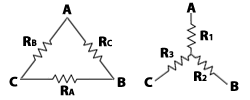Dönüşüm mantığını görelim:Yıldız olan devremize baktığımızda A ile B noktaları arasında R1 ve R2 dirençleri görülüyor. Üçgen olan devrede ise A ve B arasında Ra ve Rb dirençlerinin birbirine seri ve bu iki direncinde Rc direncine paralel olduğu görülüyor. Diğer dirençlerinde bu bağıntıları kurulduğunda aşağıdaki gibi denklemler elde ediyoruz: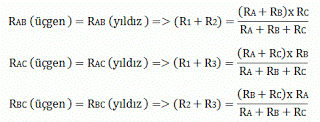Bu bağıntıları çözüp ara işlemleri yaptığımızda ise artık tam eşitlikleri elde etmiş oluyoruz.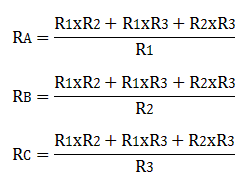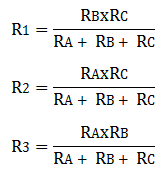Yabancı bir siteden aldığım örnekler

Örnek 1: Fig. 104 shows a number of resistances connected in delta and star. Using star/delta conversion method complete the network resistance measured between (i) Land M (ii) M and N and (iii) N and L.

Çözüm : Three resistances 12 n, 6 Ω and Ω are star connected. Transform them into delta with ends at the same points as before.Refer Fig. 105.            R12 = R1 R2 + R2 R3 + R3 R1 / R3  = 12 x 6 + 6 x 8 + 8 x 12 / 8 = 27 Ω

R23 = R1 R2 + R2R3 + R3R1 / R1 = 12 x 6 + 6 x 8 x 12 / 12 = 18 Ω

R31 = 12 x 6 + 6 x 8 x 12 / 6 = 36 Ω

Similarly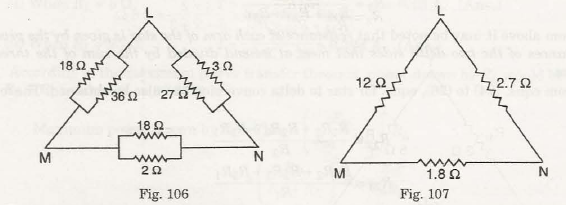Fig. 106 shows this transformed circuit connected to original delta connected resistances in the circuit 18 n, 3 Ω and 2 Ω.

Here                18 Ω and 36 Ω are in parallel;

3 Ω and 27 Ω are in parallel, and

2 Ω and 18 Ω are in parallel.

These resistances are equivalent to:

18 x 36 / 18 + 36 = 12 Ω ; 3 x 27 / 3 + 27 = 2.7 Ω and 2 x 18 / 2 + 18 = 1.8 Ω

This is shown in Fig. 107.

(i) Resistance between L and M,

RLM = 12 x (2.7 + 1.8) / 12 + (2.7 + 1.8) = 3.27 Ω

(ii) Resistance between M and N,

RMN = 1.8 x (12 + 1.8) / 1.8 + 12 + 2.7 = 1.6 Ω

(iii) Resistance between N and L,

RNL = 2.7 x (12 + 1.8) / 2.7 + (12 + 1.8) = 2.25

Örnek 2 :  In the circuit shown in Fig. 108, find the resistance between M and N.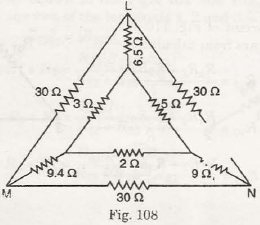Çözüm : Connecting the 1 2 3 delta [Fig. 109 (i)] to equivalent star [Fig. 109 (ii)]

R1 = R12 R31 / R12 + R23 + R31 = 5 x 3 / 5 + 2 + 3 = 1.5

R2 = R23 R12 / R12 + R23 + R31 = 2 x 5 / 5 + 2 + 3 = 1

R3 = R31 R23 / R12 + R23 + R31 = 3 x 2 / 5 + 2 + 3 = 0.6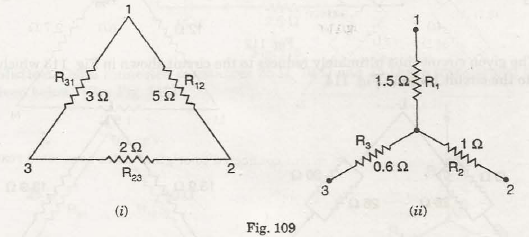Thus the original circuit reduces to that shown in Fig. 110 which further reduces to the circuit shown in Fig. 111.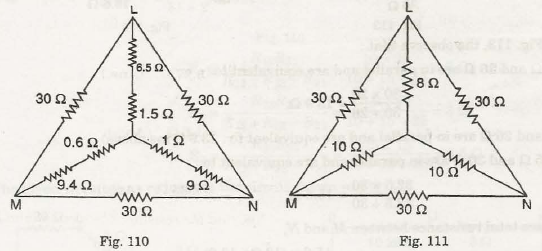Now, the inner star circuit of Fig. 110 shown as Fig. 111 is equivalent to the delta circuit

shown in Fig. 112 (ii) as appears from calculations given below :

R12 = R1 R2 + R2 R3 + R3 R1 / R3  = 8 x 10 + 10 x 10 + 10 x 8 / 10 = 26 Ω

R23 = R1 R2 + R2R3 + R3R1 / R1 = 8 x 10 + 10 x 10 x 10/ 8 = 32.5 Ω

R31 = 8 x 10 + 10 x 10 x 10 x 8 / 10 = 26 Ω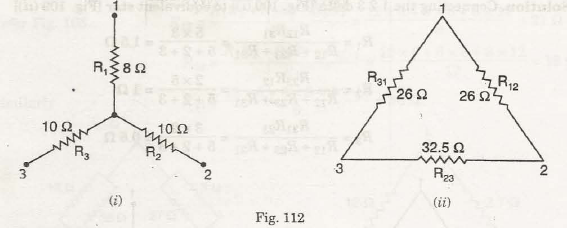The given circuit thus ultimately reduces to the circuit shown in Fig. 113 which in turn is equivalent to the circuit given in Fig. 114.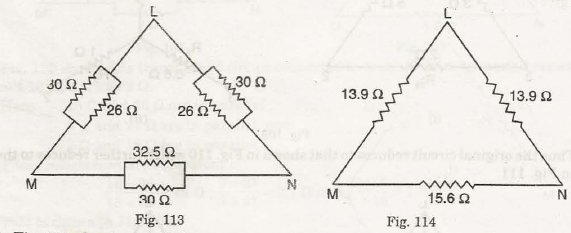In Fig. 113, the observe that :

30 Ω and 26 Ω are in parallel and are equivalent to :

30 x 26 / 30 + 26 = 13.9

30 and 26 n are in parallel and are equivalent to : 13.9 n (as above)

32.5 Ω and 30 Ω are in parallel and are equivalent to :

32.5 x 30 / 32.5 + 30 = 15.6

Hence total resistance between M and N,

RMN = 15.6 x (13.9 + 13.9) / 15.6 + (13.9 + 13.9)

= 433.69 / 43.4 = 9.99

Örnek 3 : Find the current I supplied by the battery for Fig. 115, using delta/star transformation.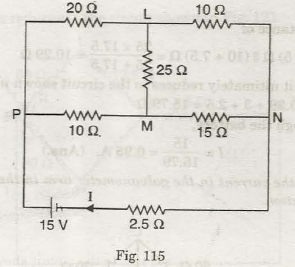Çözüm : Delta connected resistances 25 n, 10 n and 15 Ω are transformed to equivalent star as given below : (See Fig. 116)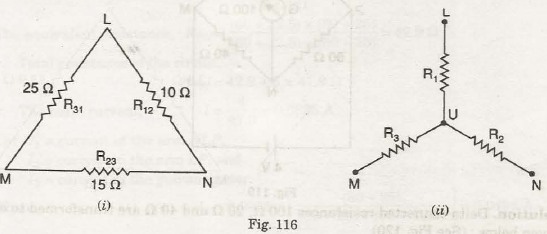R1 = R12 R31 / R12 + R23 + R31 = 10 x 25 / 10 + 15 + 25 = 5

R2 = R23 R12 / R12 + R23 + R31 = 15 x 10 / 10 + 15 + 25 = 3

R3 = R31 R23 / R12 + R23 + R31 = 25 x 15 / 10 + 15 + 25 = 7.5

The given circuit thus reduces to the circuit shown in Fig. 117.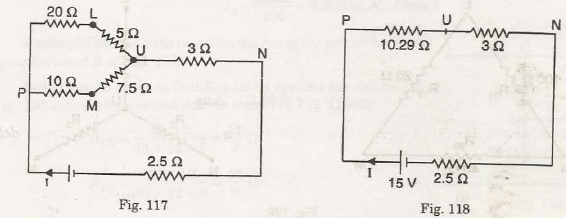The equivalent resistance of

(20 + 5) Ω || (10 + 7.5) Ω = 25 x 17.5 / 25 + 17.5 = 10.29 Ω

Thus the given circuit ultimately reduces to the circuit shown in Fig. 118.

Total resistance = 10.29 + 3 + 2.5 = 15.79 Ω

Hence current through the battery,

I = 15 / 15.79 = 0.95 A

Örnek 4 : Using Delta-star conversion find resistance between terminals AB in Fig. 124.

Çözüm :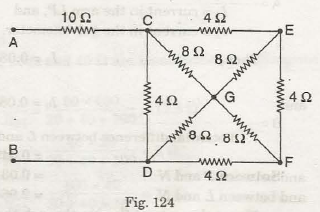• Applying delta-star conversion to CGD and EGF of Fig. 124, we get Fig. 125 (i).

• Further simplification reduces the circuit to its equivalents as in Fig. 125 (ii) and later as in Fig. 125 (iii ).

• Convert CHJ to equivalent star as in B Fig.125 (iv).

• Using series-parallel combinations, we have

RAB = (10 + 0.66) + [(0.752 + 1.6) || (2.64 + 5.6)]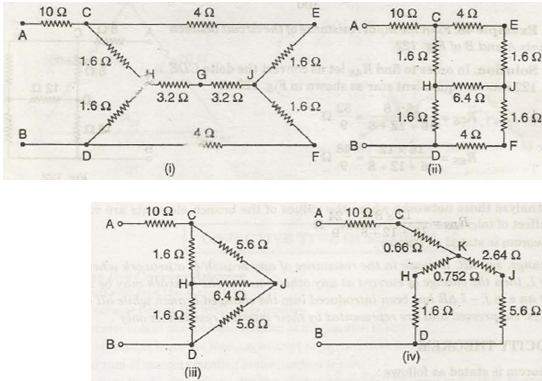= 10.66 + 2.352 x 8.24 / (2.352 + 8.24 = 12.49 Ω

Örnek 5 : Find the resistance at the A-B terminals in the electric circuit of Fig. 126, using Δ-Y transformations.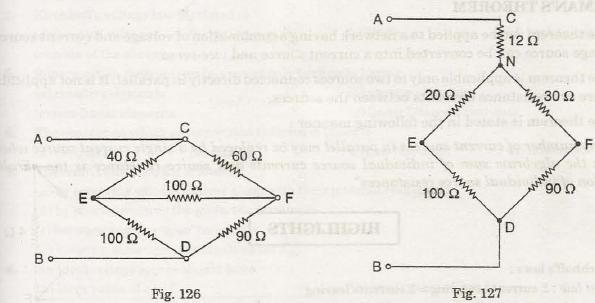Çözüm : Converting CEF into star connections, we get (Refer Fig. 127) :

CN = 60 x 40 / 60 + 40 + 100 = 24 Ω

NE = 40 x 100 / 60 + 40 + 100 = 20 Ω

NF = 60 x 100 / 60 + 40 + 100 = 30 Ω

RND = (100 + 20) x (90 x 30) / 120 + 120 = 60 Ω

RAB = 12 + 60 = 72 Ω

### Benzer Yazılar